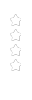# FUN WITH MATHEMAGIC

TOP BOOK ON BASIC MATH## TOP RESEARCH BOOK ON SPEED MATH / BASIC MATHAwake Mathemagician Inside You !- Can you multiply 44465 by 8888 in single line ?- Can you figure out day on 24/5/2014 in 10 seconds ?- Can you divide 123456 by 44444 instantaneously ?- Can you raise number to any integral power ?- Can you determine divisibility of 124356 by 37 just in 5 seconds ?- Can you find square root, cube root or any root of any number withoutusing calculator ?- Can you solve (2134)6 = (?)12 in 20 seconds ?SILENT FEATURES OF BOOK- Introduce VJ's universal divisibility test for all number !- Reveals unique secret behind speed mathematics !- Explain concept behind each method !- Unifies Vedic math, Trachtenberg system and modern math- Presents faster method for nth root of any number !- Gives quicker method for converting number from one base to other !- Reveal golden pattern discoveredFeatures of Golden Lemma / Golden pattern- Simplify everything right from polynomial multiplication, division , power , root , inverse- Help to build generic module in high level language to perform operation onpolynomial- Parallel multiplication architecture for multiprocessor environment- Golden pattern(process) is found and it is applicable in many area of algebra.- Golden pattern is superior over vertically crosswise pattern mentioned in Vedic math.- Improve your speed math skills.INTRODUCTIONNow–a -days speed math system ( like Vedic Mathematics , Trachtenberg System) are gaining widespread popularity among students as well as teachers. Speed math refers to faster methods and techniques to solve arithmetic calculation mentally. It saves considerable amount of time in competitive exam. So it is worthy to study speed math.In order to compute given calculation mentally, one have to recall right kind of specific method out of 1000s . Instead of doing so1. Is it possible to compute any arithmetic operation (like addition, multiplication)quickly by using scientific approach ?2. Is it possible to derive all shortcut or methods in speed math by using uniqueprinciple ?3. Is there any unique secret / principle behind speed math ?After researching speed math about 2-3 years, I realized that there is unique secret or principle behind speed mathematics !!! This book explains entire speed mathematics by using single principle and gives introduction to new number system called as global number system. It extends VM framework in some of the area like divisibility, nth root.Distinction from other Book :-It gives several new innovative tricks and goes from basic to advance math. Book also covers essential lesson in Vedic mathematics.   For more detail refer :-  https://play.google.com/store/books/details/Vitthal_B_Jadhav_Modern_Approach_to_Speed_Math_Sec?id=PXi7jCVYClAC&hl=enEssential Shortcuts

It gives essential novel shortcut useful for all competitive exam

For more detail refer :-